# R语言用lme4多层次（混合效应）广义线性模型（GLM），逻辑回归分析教育留级调查数据

## 本教程为读者提供了使用 频率学派的广义线性模型（GLM）的基本介绍。

1. 准备工作。
2. 介绍GLM。
3. 加载教育数据。
4. 数据准备。
5. 二元（伯努利）Logistic回归。
6. 二项式 Logistic 回归。
7. 多层次Logistic回归。
8. 其他族和链接函数。

– 假设检验和统计推断的基本知识。
– 回归的基本知识。
– R语言编码的基本知识。
– 进行绘图和数据处理的基本知识。

# 广义线性模型（GLM）简介

1.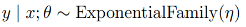，也就是y|x为指数族分布，指数族分布形式：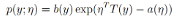2. 如果我们判断y的假设为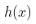，则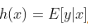3. 自然参数和输入x呈线性关系：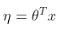# 数据准备

## 加载必要的软件包

``````# 如果你还没有安装这些包，请使用install.packages("package_name")命令。
library(lme4) # 用于多层次模型
library(tidyverse) # 用于数据处理和绘图``````

## 导入数据

``head(Edu)``

## 数据处理

``````  mutate(学校 = factor(学校),
性别 = if_else(性别 == 0, "girl", "boy"),
性别 = factor(性别, levels = c("girl", "boy")),
受过学前教育 = if_else(受过学前教育 == 0, "no", "yes"),
受过学前教育 = factor(受过学前教育, levels = c("no", "yes")))``````

## 检查缺失的数据

``  summarise_each((~sum(is.na(.))``

# 二元逻辑回归

## 探索数据：按性别和学前教育分类的留级数量

``````  group_by(性别) %>%
summarise(是否留过级 = sum(是否留过级))``````

# 构建二元逻辑回归模型

``````glm(formula ,

# 请注意，在这两张图中，Y刻度指的是留级的概率，而不是几率。概率比几率更容易解释。

``plot(Effects)``

``  predictors = list( values=c(性别boy=0, 受过学前教育yes = 0))``

# 模型评估:拟合度

## 似然比检验

``````#指定一个只有`性别'变量的模型
#使用\`anova()\`函数来运行似然比测试
anova(ModelTest, Model, test ="Chisq")``````

## AIC

Akaike信息准则（AIC）是另一个模型选择的衡量标准。与似然比检验不同，AIC的计算不仅要考虑模型的拟合度，还要考虑模型的简单性。通过这种方式，AIC处理了模型的拟合度和复杂性之间的权衡，因此，不鼓励过度拟合。较小的AIC是首选。

## 正确分类率

``````#使用\`predict()\`函数，从拟合的模型中计算出原始数据中学生的预测概率
Pred <- if_else(Pred > 0.5, 1, 0)
ConfusionMatrix <- table(Pred, TRUE)
#正确的分类率``````

## AUC（曲线下面积）

``````# 计算用该模型预测类别的AUC

AUC <- performance(Pred, measure = "auc")
AUC <- AUC@y.values\[\[1\]\]
AUC``````

AUC分数为0.60，该模型的判别能力不强。

# 二项式 Logistic 回归

## 探索数据

``````  ggplot(aes(x , y)) +
geom_smooth(method = "lm")``````

# 拟合二项式Logistic回归模型

``````glm(cbind(是否留过级, TOTAL-是否留过级) ~ 学校平均社会经济地位,
family = binomial(logit))``````

# 解释

``````#注意，为了对二项回归模型使用summ()函数，我们需要将结果变量作为对象。

``plot(allEffects)``

# 多层次二元逻辑回归

`````` group_by(学校) %>%
summarise(PROP = sum(是否留过级)/n()) %>%
plot()``````

# 我们还可以通过学校来绘制性别和留级之间的关系，以了解性别和留级之间的关系是否因学校而异。

``````mutate(性别 = if_else(性别 == "boy", 1, 0)) %>%
ggplot(aes(x = 性别, y = 是否留过级, color = as.factor(学校))) +``````

`````` mutate(性别 = if_else(性别 == "girl", 0, 1),
受过学前教育 = if_else(受过学前教育 == "yes", 1, 0)) %>%
group_by(学校) %>%
mutate(性别 = 性别 - mean(性别),``````

# 中心化变量

``````        受过学前教育 = if_else(受过学前教育 == "yes", 1, 0)) %>%
group_by(学校) %>%
mutate(性别 = 性别 - mean(性别),
受过学前教育 = 受过学前教育 - mean(受过学前教育)) %>%
ungroup() %>%``````

## 只有截距模型

``````glmer(是否留过级 ~ 1 + (1|学校),
family = binomial(logit),
optCtrl = list(maxfun=2e5))``````

0.33的ICC意味着结果变量的33%的变化可以被数据的聚类结构所解释。因此，多层次模型的使用是必要的，也是有保证的。

# 完整模型

`` glmer(是否留过级 ~ 性别 + 受过学前教育 + 学校平均社会经济地位 + (1 + 性别 + 受过学前教育|学校)``

# 这提供了证据表明，与非多层次模型相比，多层次模型可能会对模型的估计产生影响。

``sum(Model_Full)``

``plot((Model)``

`` glmer(是否留过级 ~ 性别 + 受过学前教育 + 学校平均社会经济地位 + (1 + 受过学前教育|学校),``
``````#拟合一个不完整的模型，剔除`受过学前教育'的随机斜率项
glmer(是否留过级 ~ 性别 + 受过学前教育 + 学校平均社会经济地位 + (1 + 性别|学校),``````

## AIC

``````AIC #full模型
AIC#＃没有性别的模型
AIC #＃没有受过学前教育的模型
AIC#＃没有随机斜率的模型``````

# 其他族（分布）和链接函数

## 参考文献

Bates, D., Maechler, M., Bolker, B., & Walker, S. (2015). _Fitting Linear Mixed-Effects Models Using lme4. Journal of Statistical Software, 67_(1), 1-48. doi:10.18637/jss.v067.i01

Enders, C. K., & Tofighi, D. (2007). Centering predictor variables in cross-sectional multilevel models: A new look at an old issue. _Psychological Methods, 12_(2), 121-138. doi:10.1037/1082-989X.12.2.121

Kaizong Ye拓端研究室（TRL）的研究员。

​非常感谢您阅读本文，如需帮助请联系我们！QQ在线咨询

15121130882

0571-63341498

## 关注有关新文章的微信公众号

This will close in 0 seconds